Next: FINDING THE SHORTEST AND Up: thesis Previous: PATHWAY SUMS FOR CHAIN   Contents

# TOTAL ESCAPE PROBABILITY FOR CHAIN GRAPHS, #MATH971#

So long as there is at least one escape route from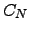the total escape probability must be unity: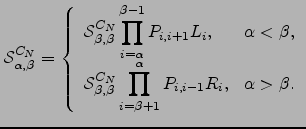(D.1)

otherwise, if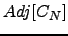is the empty set, we have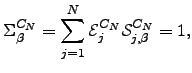(D.2)

For example, to show that the formulae in Appendix C are consistent with the first result we expand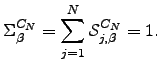(D.3)

and define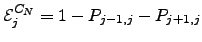(D.4)

for convenience, so that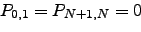(D.5)

Using the recursion relations in Equation C.2 (which assume that there is an escape route from) we can show that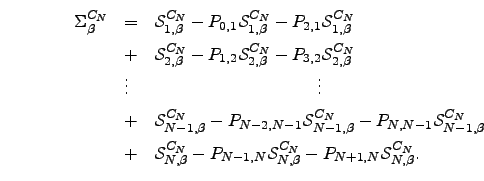(D.6)

for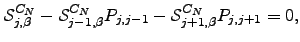. We can now group together terms in Equation D.5 into sets of three that sum to zero. The terms that do not immediately cancel are as follows. From the first and second lines of Equation D.5 we have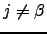(D.7)

because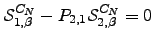(D.8)

Similarly, on the last two lines we find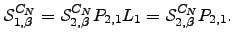(D.9)

because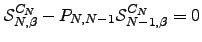(D.10)

The final remaining terms are: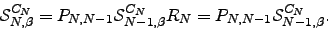(D.11)

which proves Equation D.1.Next: FINDING THE SHORTEST AND Up: thesis Previous: PATHWAY SUMS FOR CHAIN   Contents
Semen A Trygubenko 2006-04-10